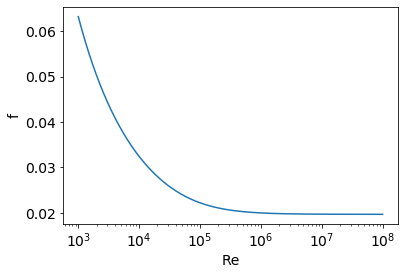Solving equations¶

In :
import numpy as np
import matplotlib.pyplot as plt
%matplotlib inline
from scipy.optimize import fsolve

Review: linear equations¶

To solve a system $Ax=b$ for x, we do the following:

• Define the matrix $A$ and the array $b$.
• Use x = np.linalg.solve(A,b)

Example

Solve $$\left[ \begin{array}[ccccc] --2 & 1 & 0 & 0 & 0 \\ 1 & -2 & 1 & 0 & 0 \\ 0 & 1 & -2& 1 & 0 \\ 0 & 0 & 1 & -2& 1 \\ 0 & 0 & 0 & 1 & -2 \end{array} \right]\cdot \left[ \begin{array}[c] \\x_0\\x_1\\x_2\\x_2\\x_4\end{array}\right] = \left[\begin{array}[c] \\1\\1\\1\\1\\1\end{array}\right]$$

In :
A = np.array([[-2,1,0,0,0],[1,-2,1,0,0],[0,1,-2,1,0],[0,0,1,-2,1],[0,0,0,1,-2]])
b = np.ones(5)

x = np.linalg.solve(A,b)
x
Out:
array([-2.5, -4. , -4.5, -4. , -2.5])

Here's a link to more linear algebra operations.

Nonlinear equations¶

Recall¶

• What are the key elements to solving nonlinear equations?
• List the parts, the form, and things you need.

Exercise¶

• Before learning the details about solving equations using Python, see if you can guess how to solve the following equation using Python's solver fsolve, based on what you need to solve equations: $$f(x) = x^2-5.$$
In :
from scipy.optimize import fsolve

def f(x):
return x**2 - 5

x_guess = 1

x = fsolve(f, x_guess)
x
Out:
array([2.23606798])

Solving a nonlinear equation involves first putting it in the form $f(x)=0$. We know the function $f$ and we want to find the value of $x$ that gives $f(x)=0$.

To solve this, do the following:

• Define the function f(x)
• Set an initial guess for x.
• We need to import a library: from scipy.optimize import fsolve
• Call the fsolve function: x = fsolve(f, x0)
• f is the name of the function you are solving, and x0 is your initial guess.

Exercise¶

Solve for both roots of $$f(x) = x^2-5.$$

In :
# the problem is solved above. Here's the solution using an anonymous function:

fsolve(lambda x: x**2 - 5, x_guess)
Out:
array([2.23606798])

Multiple equations in multiple unknowns¶

• What do we do if we are solving multiple equations in multiple unknowns?
• For example, suppose we have $h(y,z)=0$ and $g(y,z)=0$. Such as: $$h(y,z) = y+2z,$$ $$g(y,z) = \sin(y)/z.$$

• Do you think Python's fsolve function can handle this?

• How will you go about specifying the problem?
• What if there were three equations in three unknowns? Four and four? etc.?
• If you were coding fsolve how would you account for all these possibilities?

General approach¶

• Instead of $f(x)=0$, we solve
• $\vec{f}(\vec{x})=\vec{0}$.
• That is, $\vec{x}$ is a vector of unknowns, and $\vec{f}$ is a vector of functions.
• $\vec{f}(\vec{x})$ takes a vector $\vec{x}$, and returns a vector $\vec{f}$.

Details¶

• Consider again the two functions for the two unknowns: $h(y,z) = 0$, and $g(y,z)=0$. $$h(y,z)=y+2z,$$ $$g(y,z) = \sin(y)/z$$
• For computer solvers, we write using a generic, standard notation.
• **We want all solvers to look like $f(x)=0.$**
• We would write these as $$f_0(x_0,x_1) = x_0+2x_1,$$ $$f_1(x_0,x_1) = \sin(x_0)/x_1,$$ which becomes: $$\left[\begin{matrix} f_0(x_0,x_1) \\ f_1(x_0,x_1)\end{matrix}\right] = \left[\begin{matrix}x_0+2x_1 \\ \sin(x_0)/x_1\end{matrix}\right] = \left[\begin{matrix}0 \\ 0\end{matrix}\right],$$ or $$\vec{f}(\vec{x}) = \left[\begin{matrix}x_0+2x_1 \\ \sin(x_0)/x_1\end{matrix}\right] = \vec{0},$$ that is, $$\vec{f}(\vec{x})=\vec{0}.$$

• We solve the system just like before:

• Just define the vector function. It takes an array of unknowns $\vec{x}$, and returns an array of function values $\vec{f}$.
• We use the same solver, but we give an array of initial guesses $\vec{x}_0$.

Note Here is a link to more information about the fsolve function with extra arguments that can give more control over the solution process.

Exercise¶

• Solve the following two equations in two unknowns. $$h(y,z)=y+2z,$$ $$g(y,z) = \sin(y)/z.$$
• Use $y_0=1$, and $z_0=2$ as an initial guess.
In :
def hg(yz):
y = yz
z = yz

h = y + 2*z
g = np.sin(y)/z

return np.array([h,g])

#---------------

yz_guess = np.array([1,2])

yz = fsolve(hg, yz_guess)

print("yz =", yz, "hg =", hg(yz))
yz = [ 9.42477796 -4.71238898] hg = [0.00000000e+00 4.82244674e-15]

Excercise¶

Solve the following system of three equations in three unknowns:

$$x^2 + y^2 = 1,$$$$xy + yz = -1.1,$$$$y^2 + z^2 = 2.$$

A reasonable guess for all variables is $x=y=z=2.$

In :
def f(xyz):
x = xyz
y = xyz
z = xyz

f0 = x**2 + y**2 - 1
f1 = x*y + y*z + 1.1
f2 = y**2 + z**2 - 2

return np.array([f0, f1, f2])

#------------

xyz_guess = np.array([2.0, 2.0, 2.0])

xyz = fsolve(f, xyz_guess)

print(xyz)
[ 0.10056089 -0.99493091  1.00504353]

Exercise¶

The Colbrook equation is used to compute pressure drops across pipes, and therefore to compute pumping requirements and costs.

The Colebrook equation is given by $$\frac{1}{\sqrt{f}} = -2\log_{10}\left(\frac{\epsilon/D}{3.7}+\frac{2.51}{Re\sqrt{f}}\right),$$ or, $$F(f, Re, \epsilon/D) = \frac{1}{\sqrt{f}} + 2\log_{10}\left(\frac{\epsilon/D}{3.7}+\frac{2.51}{Re\sqrt{f}}\right)=0.$$

• Solve the Colbrook equation for $f$, for each $Re$ listed below, for the given single value of $\epsilon/D$.
• Plot the result of $f(Re)$ with $Re$ on a log scale.

Approach

• Start simple, then add more functionality.
• Verify results at each step.
• Question: what are the different parts required?
In :
Re     = np.logspace(3,8,1000)
epsD   = 0.001
fguess = 0.01

def F(f, Re, epsD):
return 1/np.sqrt(f) + 2*np.log10(epsD/3.7 + 2.51/Re/np.sqrt(f))

# first, just get it to work with just one Re

f = fsolve(F, fguess, args=(5000, epsD))
print("for Re=5000, f =", f)

# now, make an array of f and fill in each value in a loop:

f = np.empty(len(Re))
for i in range(len(f)):
f[i] = fsolve(F, fguess, args=(Re[i], epsD))   # note the Re[i]

#--------- plot results

plt.rc('font', size=14)
plt.semilogx(Re, f)
plt.xlabel('Re')
plt.ylabel('f');
for Re=5000, f = [0.03849536]In [ ]: